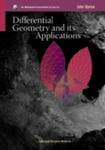## Scholarship Collection#### Title

Differential Geometry and its Applications

#### Description

Differential geometry has a wide range of applications, going far beyond strictly mathematical pursuits to include architecture, engineering, and just about every scientific discipline. John Oprea's second edition of Differential Geometry and Its Applications illuminates a wide range of ideas that can be beneficial to students majoring not only in mathematics but also in other fields. Differential Geometry and Its Applications was written to help students adapt to "a type of mathematics that is a unified whole," one that mixes together geometry, calculus, linear algebra, differential equations, complex variables, the calculus of variations, and various notions from the sciences. The textbook touches on many different mathematical concepts, including aspects of linear algebra, the Gauss-Bonnet Theorem, and geodesics. It also encourages students to visualize and experiment with the ideas they are studying through their use of the computer program Maple. This allows students to develop a better understanding of the mathematics involved in differential geometry. The book is full of exercises that challenge students to combine concepts from different areas of mathematics to obtain solutions.

#### ISBN

978-0-88385-748-9

7-10-2007

#### Publisher

Mathematical Association of America

Washington D. C.

#### Disciplines

Algebraic Geometry | MathematicsCOinS